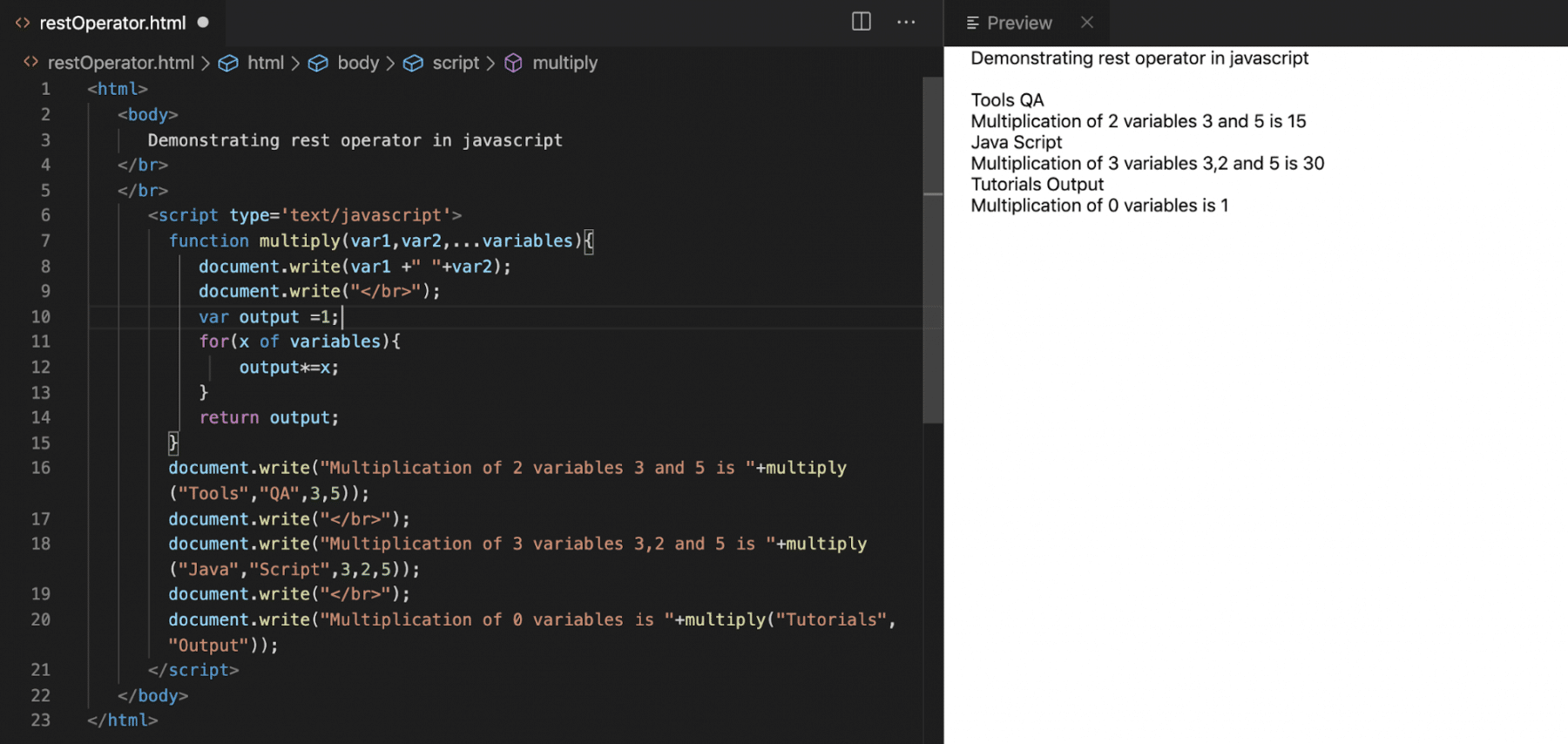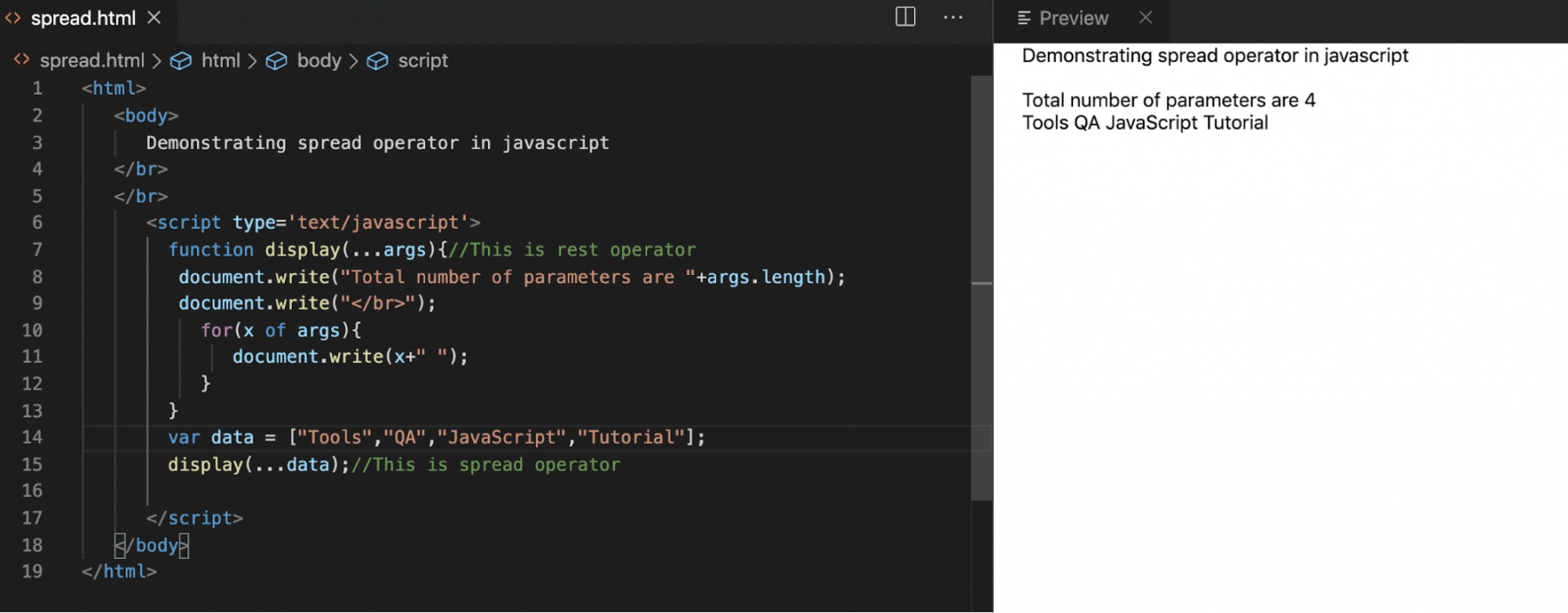All the programming languages provide different ways to pass an arbitrary, indefinite number of parameters to a function. Javascript has also provided concepts that make the "passing of arbitrary parameters" to a function very easy. In this article, we are going to understand how to handle the arbitrary parameters using the "rest parameter" in JavaScript. We will also concentrate on below items to understand the concepts of handling the arbitrary parameters:

• How to handle arbitrary parameters using the "arguments" variable in JavaScript?
• We will also understand how to handle the arbitrary parameters using the "rest parameter" in JavaScript?
• How to expand iterable objects using the "spread operator" in JavaScript?

## How to handle arbitrary parameters using the "arguments" variable in JavaScript?

Arguments are a special type of inbuilt variable available in JavaScript, which can handle an arbitrary number of parameters in a function. Consider a scenario where the function definition doesn't accept any parameters, but at run time, the caller wants to pass some parameters. Such kind of parameters can be accessed in the function definition using the "arguments" variable. One can access the arguments with the help of the index of the arguments variable.

Let's understand the usage of "arguments" with the help of the following example:

Example:

``````<html>

<body>

Demonstrating arguments keyword in javascript </br> </br>

<script type='text/javascript'>

function display(){
document.write(arguments + " " + arguments);
}

display("Tools","QA");

</script>

</body>

</html>
``````

Save the file with name  arguments.html and open it in any browser (Chrome, Firefox, or IE). It should show the output asIn the above example, We can see that the display method doesn’t have any parameters. Still, while calling, we are sending two parameters, and we can access it by using the "arguments" variable with the help of the index.

Note: Even though the "arguments" variable is both array-like and iterable, it’s not an array. Additionally, it does not support array methods, so we can’t call arguments.map(...) for example.

## How to handle arbitrary parameters using the "rest parameter" in JavaScript?

The "rest parameter" is the advanced way provided by JavaScript to handle the arbitrary number of parameters. Its syntax looks like below:

Syntax:

``````function functionName(...args){

//Statements need to get executed

}
``````

Let's try to understand the details and usage of the rest parameter with the help of the following example:

Consider a case where we need to perform a multiplication operation; the function will look like:

``````function multiply(variable1, variable2){

return variable1*variable2;

}
``````

Now, the above code perfectly works when there are only 2 arguments, But imagine there is a situation where we need to multiply n number of variables, and n can be different for each time one invokes the function. In this case, the number of arguments entirely depends on the caller.

To achieve this, we can use the rest parameter, as shown below:

Example: when the function just accepts the "rest" parameter

``````<html>

<body>

Demonstrating rest operator in javascript

</br>

</br>

<script type='text/javascript'>

function multiply(...variables){

var output =1;

for(x of variables){

output*=x;

}

return output;

}

document.write("Multiplication of 2 variables 3 and 5 is "+multiply(3,5));

document.write("</br>");

document.write("Multiplication of 3 variables 3,2 and 5 is "+multiply(3,2,5));

document.write("</br>");

document.write("Multiplication of 0 variables is "+multiply());

</script>

</body>

</html>
``````

Save the file with name  restOperator.html and open it in any browser (Chrome, Firefox, or IE). It should show the output asIn the above example, we can see that there is only one multiply function, but it can take n number of variables, and the caller will decide these variables. The "rest" parameter is being specified by the "...variables" in the multiply method, and it can accept zero or more parameters.

The "rest" parameter can be combined with other parameters of the function as well, but it will always be the last parameter of the function. Let's understand the same with the help of the following example:

Example: when the function accepts named parameters with the rest parameter

Let's consider an example that takes two mandatory parameters, and the last variable is a "rest" parameter. The following code snippet shows the usage of the "rest" parameter as the last parameter of the function:

``````<html>

<body>

Demonstrating rest operator in javascript

</br>

</br>

<script type='text/javascript'>

function multiply(var1,var2,...variables){

document.write(var1 +" "+var2);

document.write("</br>");

var output =1;

for(x of variables){

output*=x;

}

return output;

}

document.write("Multiplication of 2 variables 3 and 5 is "+multiply("Tools","QA",3,5));

document.write("</br>");

document.write("Multiplication of 3 variables 3,2 and 5 is "+multiply("Java","Script",3,2,5));

document.write("</br>");

document.write("Multiplication of 0 variables is "+multiply("Tutorials","Output"));

</script>

</body>

</html>
``````

Save the file with name  restOperator.html and open it in any browser (Chrome, Firefox, or IE). It should show the output asAs we can see in the above code snippet, the multiple functions are accepting var1 and var2 as mandatory parameters, and the last parameter "...variables" is the "rest" parameter. The user can invoke the function by passing the two necessary parameters and the rest parameters as any arbitrary number of parameters.

## How to expand iterable objects using the "spread operator" in JavaScript?

Till now, we have seen how to get an array from the list of parameters. But sometimes we need to do precisely the reverse.

For example, the built-in function Math.max that returns the greatest number from a list:

``````Math.max(3, 5, 1) // Returns 5
``````

Now let’s say we have an array [3,5,1]. How do we call "Math.max" with it? Passing it “as is” won’t work, because "Math.max" expects a list of numeric arguments and not a single array object:

``````let arr = [3, 5, 1];

Math.max(arr); // NaN
``````

And surely we can’t manually list items in the code Math.max(arr, arr, arr), because we may be unsure how many parameters are there. When the function invokes, there could be a lot, or there could be no parameters.

The spread syntax can handle such a scenario. It appears similar to rest parameters, also uses "..." but does quite the opposite. When "...arr" is used in the function call, it “expands” an iterable object "arr" into the list of arguments. Its syntax looks like below:

Syntax:

``````var array=[va1,val2,val3…,valN];

callingFunction(...array); //...variablename convert array to list of arguments
``````

For "Math.max" it will look like:

``````let arr = [3, 5, 1];

Math.max(...arr); // 5 (spread turns array into a list of arguments)
``````

We also can pass multiple iterable objects this way:

``````let arr1 = [1, -2, 3, 4];
let arr2 = [8, 3, -8, 1];

Math.max(...arr1, ...arr2); // 8
``````

We can even combine/ join the spread syntax with normal values:

``````let arr1 = [1, -2, 3, 4];
let arr2 = [8, 3, -8, 1];

Math.max(1, ...arr1, 2, ...arr2, 25); // 25
``````

Let's understand the detailed usage of the "spread" syntax with the help of the following example:

Example:

``````<html>

<body>

</br>

</br>

<script type='text/javascript'>

function display(...args){//This is rest operator

document.write("Total number of parameters are "+args.length);

document.write("</br>");

for(x of args){

document.write(x+" ");

}

}

var data = ["Tools","QA","JavaScript","Tutorial"];

</script>

</body>

</html>

``````

Save the file with name  spread.html and open it in any browser (Chrome, Firefox, or IE). It should show the output asAs we can see in the above example,  we are passing data array to the "display" function with the help of the spread operator, and the data array converts to a list of arguments.

## Key Takeaways

• The "arguments" is a special array-like object that contains all the parameters passed by their index.
• The "rest" parameters pass and handle an arbitrary number of arguments.
• The "spread" operator is used to convert the iterable objects like an array to a list of independent parameters.

Let's move to the next article, where we will cover the details of the object-oriented concept Classes in JavaScript.